Vibrational Modes and Spectrum of a 2d Spring Model in a Magnetic Field

Taylor L. Hughes March 1, 2008

(Submitted as coursework for Appied Physics 372, Stanford University, Winter 2008)

Systems of coupled springs are the natural paradigm for many phenomena in quantum field theory and many-particle physics. It is often useful to study these problems to gain better physical intuition for the behavior of field-theoretical models which are presented in a more difficult context. Here we shall study one such problem: a 2d lattice of charged particles coupled to their nearest-neighbors with a spring. We will analyze the local spectral function with both open and periodic boundary conditions as well as the full global spectrum. Then we will add in a magnetic field coupled to the charged particles and make some simple comparisons with the zero field case.

Classical Spring Problem and the Green's Function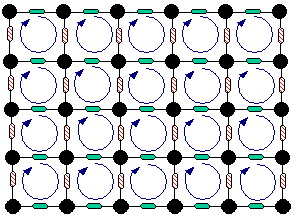Let us consider the lattice given above. The sites are particles with charge e and the lattice links represent springs with different spring constants Kx, Ky. The circulating arrows represent a magnetic field B with a direction, in this case, into the page. Let N be the number of sites along both the x and y directions so that the total number of lattice sites is N2. For simplicity I will set the charge and the mass of all the particles to 1. So in principle, without considering the boundary conditions the model is parameterized only by B, Kx, and Ky . In addition to these I will add two more parameters a and b which are real numbers between 0 and 1 and indicate the strength of the coupling between sites 1 and N in the x and y directions respectively. For example, when a=0 the x direction is an open boundary, and when, say, b=1 the y direction has periodic boundary conditions. This will allow us to explore the effect of boundary conditions on the spectrum.

To study this problem we will examine the properties of the Green's function of this system. We will first write down the equations of motion in dynamical matrix form and then invert them to get the Green's function as a function of frequency and position. Once we have the Green's function we will extract the vibrational spectrum. To begin, we will write down the coupled equations of motion: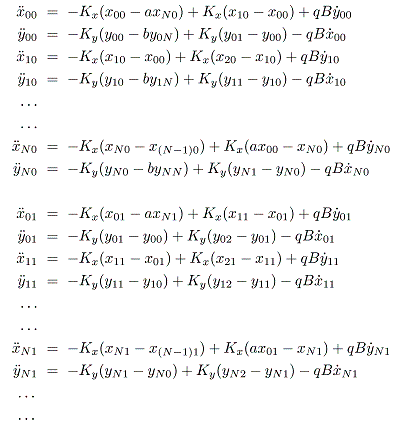where the two subscripts on each coordinate indicate the x and y lattice index. Note that the terms which are coupled across the end of each horizontal chain have factors of a and those coupled across the end of each vertical chain have a factor of b. This effectively let's us change the boundary conditions by varying these two parameters as we stated earlier. Additionally, the x and y coordinates are not independent in the presence of a magnetic field. This is a result of the Lorentz force: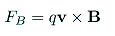where v is the particle velocity. Everything is linear in time in this problem so we can transform this differential equation into a simple frequency dependent matrix equation by Fourier transform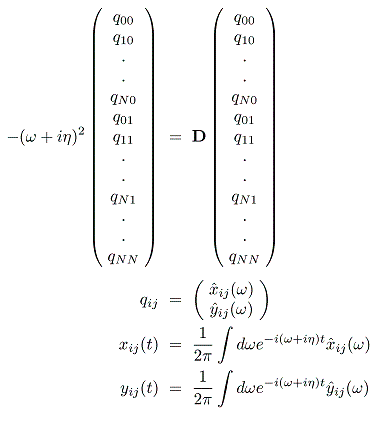The matrix D is the dynamical matrix and is block tri-diagonal with blocks of size 2N x 2N. The total size of D is 2N2 x 2N2 which gets large very rapidly. The Green's function for this dynamical matrix is defined as: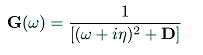There are tricks for obtaining the Green's function efficiently, especially if one only needs part of the matrix, but since we don't anticipate the need for huge lattices for our illustrative purposes we chose to use a brute force inversion method that solves for the entire Green's function matrix. This also gives us the ability to study spatial properties of the entire system. We are interested in the spectral function so we will actually calculate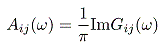Calculations and Results

First we will turn off the magnetic field and plot the trace of the spectral function vs. frequency as well as some examples of the vibrational modes in a 2d intensity plot. The intensity plots will show the strength of the spectral weight concentrated at each lattice site at a particular frequency. Additionally, for the intensity plots we ignore any effects of mode polarizations and simply trace over the local x and y degrees of freedom. For all calculations I have used a frequency resolution of 0.005 and an η of 0.01

Calculations for Kx=Ky=1.5,B=0, a=b=1.0, and N=12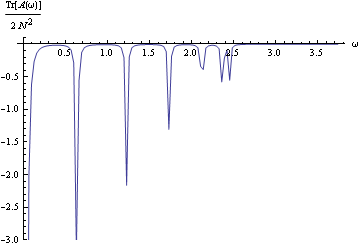For this case I only show the full spectrum and not the local intensity plots. Because of translation invariance the local intensity plots won't show any interesting features. The spectrum cuts off once the frequency is near 3 which shows the presence of a finite bandwidth. The spectrum also shows some well defined modes which should be very degenerate.

Calculations for Kx=1.0,Ky=1.5,B=0, a=0.0, b=1.0, and N=12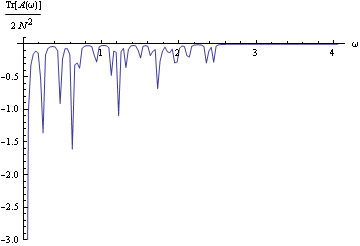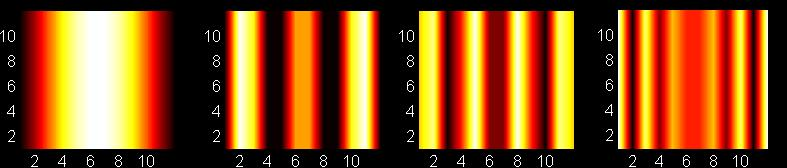In the spectrum plot it is clear that the modes that were once degenerate in the symmetric case have now split up into many separate frequencies. The intensity plots, which increase in energy from left to right, clearly show the explicit broken 90 degree rotation symmetry. They do exhibit the remaining 180 degree rotation symmetry and a trend for an increasing number of oscillations as the energy increases.

Calculations for Kx=1.5,Ky=1.5,B=3.2, a=0.0, b=1.0, and N=12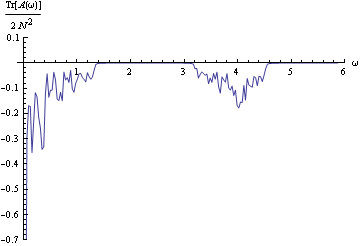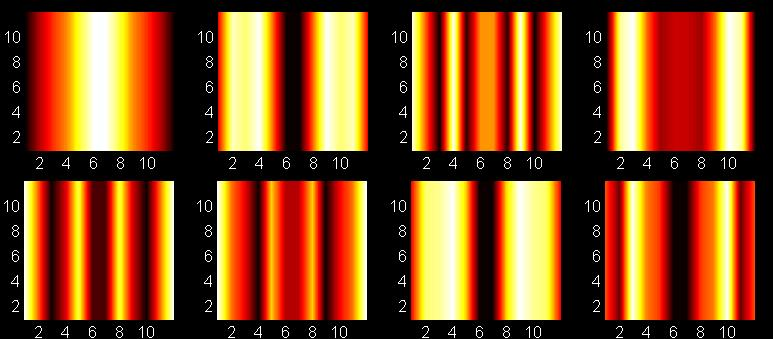For a strong B field something interesting happens in the spectrum. We effectively have two continua of states separated by an energy gap. The states in the lower portion cut-off around ω =1.5 and the second set begin around ω =3.2. The low energy modes are most likely just the typical oscillator modes we have seen before. However, the higher energy modes only turn on once the magnetic field frequency has been reached. These modes be a combination of the normal oscillator modes on top of some magnetic oscillation. In the intensity plot grid frequency increases from left to right and the first and second rows are modes in the low and high energy regions respectively. We can see how the high-energy threshold scales as we increase magnetic field. For B=5.0 we get the spectrum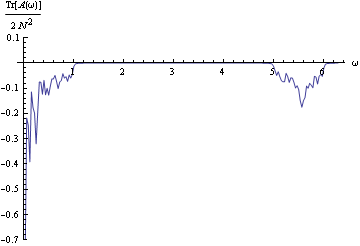It is clear from this spectrum that again the threshold is at the magnetic energy scale so this supports our earlier conclusions.

Calculations for Kx=50.0,Ky=50.0,B=0.1, a=0.0, b=0.0, and N=12

Finally, we will try to isolate some magnetic oscillation modes. We turn up the bulk oscillator strength extremely high so that it will take a very large energy to excite any of these modes. We also set the system to have open boundary conditions along each direction.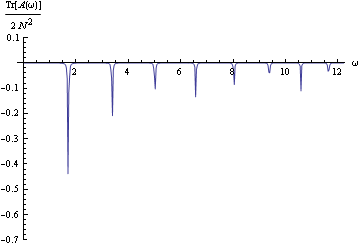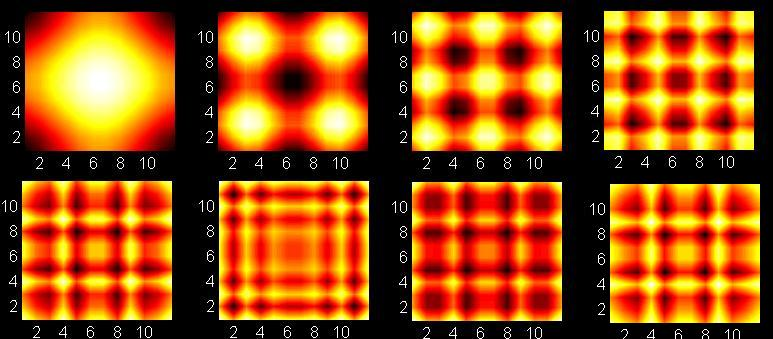The spectrum here also shows some interesting features. Instead of a continuum of states we now have sharp peaks associated to the modes. The intensity plots show the spatial distribution of the spectral weight near first eight peaks with frequency increasing to the right. These modes have an interesting spatial structure and are again 90-degree rotation symmetric since we have the same boundary conditions on the x and y directions. The spatial structure of the modes

Source Code

The source code for all of the calculations done in this work can be found here. We used the GNU scientific library for the matrix inversion routine and complex numbers. This library is standard in all GNU Linux distributions and can be used on any platform including Windows via theCygwin emulator. Typical execution times for 200 frequency points and N=12 were a few minutes.

© 2008 T. L. Hughes. The author grants permission to copy, distribute and display this work in unaltered form, with attribution to the author, for noncommercial purposes only. All other rights, including commercial rights, are reserved to the author.